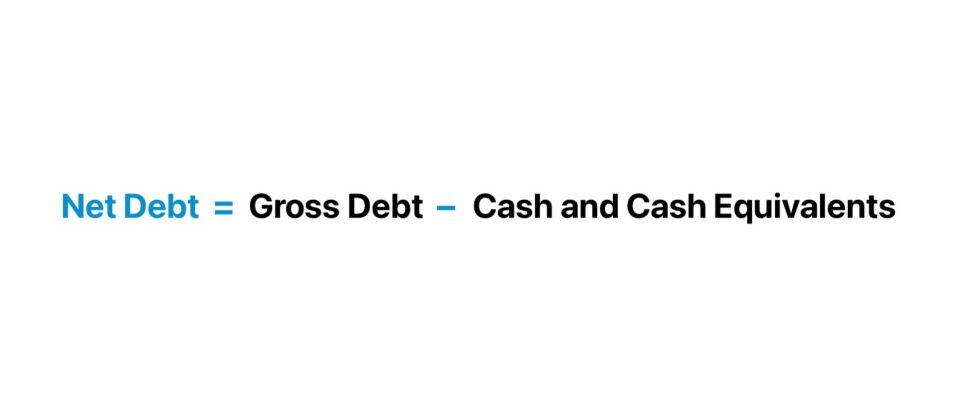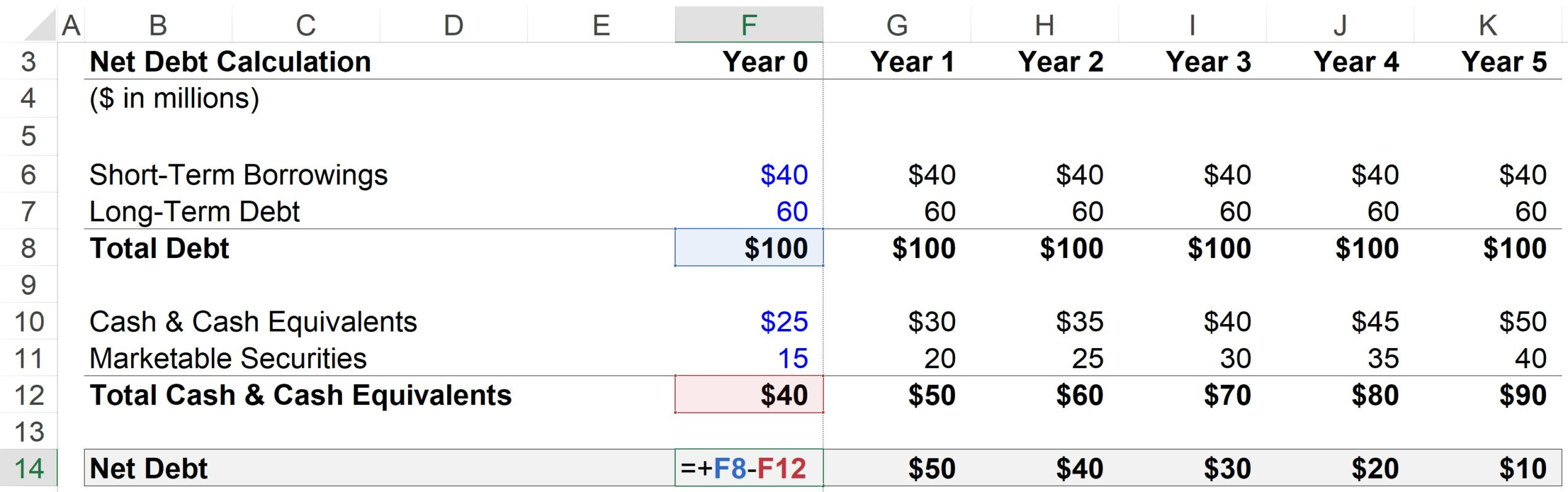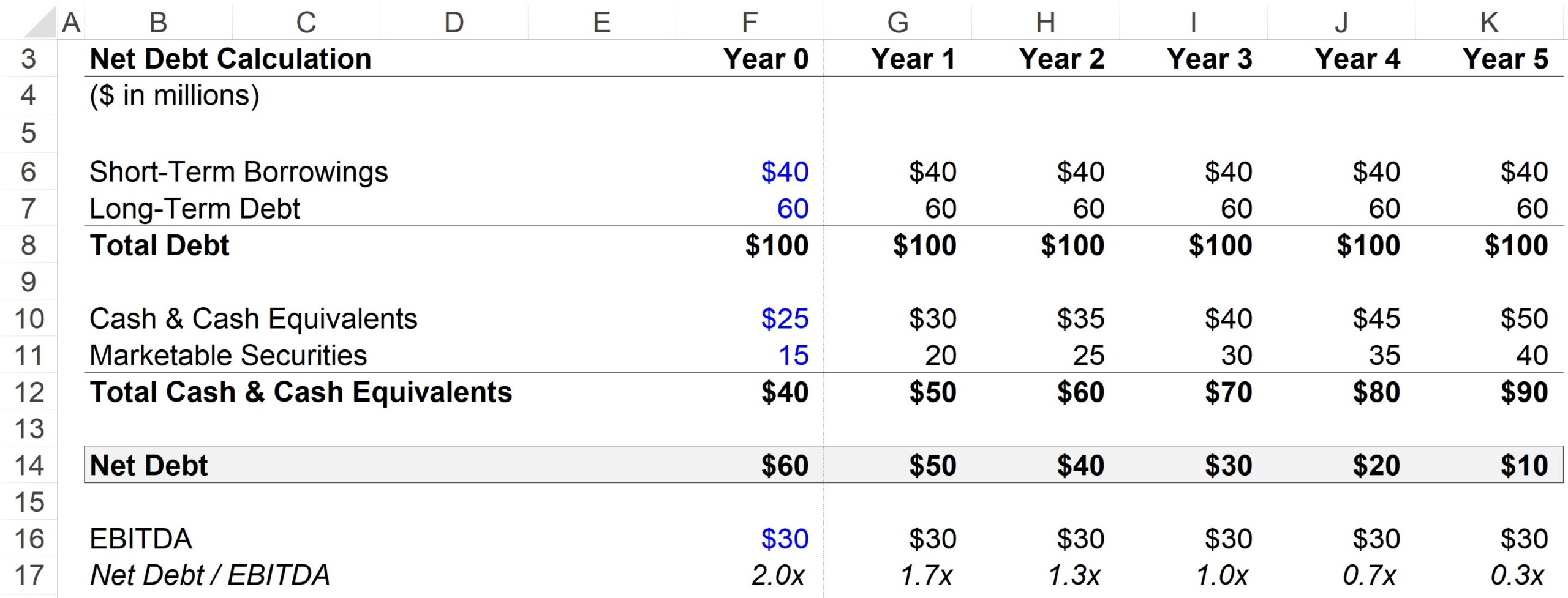Welcome to Wall Street Prep! Use code at checkout for 15% off.# Net Debt

Guide to Understanding the Net Debt Concept## How to Calculate Net Debt?

The net debt of a company represents the remaining debt balance once the company’s cash is used to help pay down as much debt as possible.

Frequently used to determine the liquidity of a company, the metric shows the remaining debt balance if all of a company’s cash and cash equivalents were hypothetically used to pay down its outstanding debt obligations.

The underlying idea behind net debt is that the cash sitting on a company’s balance sheet could hypothetically be used to pay down outstanding debt if necessary.

Since the assumption is that cash helps offset the debt burden, the value of a company’s cash and cash equivalents are deducted from the gross debt.

Calculating a company’s net debt balance consists of three steps:

1. Calculate the Sum of All Debt and Interest-Bearing Obligations
2. Calculate the Sum of Cash and Cash Equivalents (e.g. Marketable Securities)
3. Subtract Gross Debt by Cash and Cash Equivalents

## Net Debt Formula

The formula for calculating net debt is as follows.

Net Debt = Gross DebtCash and Cash Equivalents

Where:

• Gross Debt → Comprises all short-term and long-term debt obligations, such as short-term and long-term loans and bonds — as well as financial claims such as preferred stock and non-controlling interests.
• Cash and Cash Equivalents → Contains all cash and highly liquid investments — which refer to short-term holdings such as marketable securities, money market funds, and commercial paper.

## What is a Good Net Debt?

If the net debt of a company is negative, this suggests the company has a significant amount of cash and cash equivalents on its balance sheet.

The negative balance could be an indication the company is not financed with an excessive amount of debt.

In contrast, it could also just mean the company is holding onto more cash in comparison to debt (e.g. Microsoft, Apple).

Given a negative net balance, the enterprise value of these companies will be lower than their equity value. Recall that the enterprise value represents the value of a company’s operations – which excludes any non-operating assets.

Therefore, companies that have accumulated large cash reserves will have a higher equity value than enterprise value.

## Net Debt Calculator

We’ll now move to a modeling exercise, which you can access by filling out the form below.Submitting...

## 1. Operating Assumptions

Suppose we’re tasked with calculating the net debt of a hypothetical company given the following financials in Year 0.

Year 0 Financials

• Short-Term Borrowings = \$40m
• Long-Term Debt = \$60m
• Cash & Cash Equivalents = \$25m
• Marketable Securities = \$15m

For each period in the forecast, all debt and debt-equivalents are assumed to remain constant. Cash and marketable securities, on the other hand, are going to grow by \$5m per year.

• Step Function, Debt = Constant (“Straight-Line”)
• Step Function, Cash = +\$5 per year

Given the growth in cash and cash equivalents, while the debt amount remains constant, it would be reasonable to expect the company’s net debt to decrease each year.

## 2. Net Debt Calculation Example

For Year 1, the steps to compute net debt are as follows:

1. Total Debt = \$40m Short-Term Borrowings + \$60m Long-Term Debt = \$100m
2. Less: Cash & Cash Equivalents = \$30m Cash + \$20m Marketable Securities
3. Net Debt = \$100m in Total Debt – \$50m Cash & Cash Equivalents = \$50m

Therefore, the net debt balance in Year 1 is \$50 million.## 3. Net Debt to EBITDA Ratio Calculation Example

A common leverage ratio is the net debt-to-EBITDA ratio, which divides a company’s total debt minus cash balance by a cash-flow metric, which is EBITDA in this case.

For our EBITDA assumption, we’ll be using \$30m for each period in the forecast.

Since cash can be used to pay down debt, many leverage ratios use net rather than gross debt, as one could argue that net (not gross) debt is a more accurate representation of the company’s actual leverage.

From the completed output below, we can see how the net debt-to-EBITDA ratio declines from 2.0x in Year 0 to 0.3x by the end of Year 5, which is driven by the accumulation of highly liquid, cash-like assets.

But in the same time span, our total debt / EBITDA ratio remains constant at 3.3x as it does not take into account the growth in cash and cash equivalents.Step-by-Step Online Course

### Everything You Need To Master Financial Modeling

Enroll in The Premium Package: Learn Financial Statement Modeling, DCF, M&A, LBO and Comps. The same training program used at top investment banks.

Inline Feedbacks
Alex Shan
May 17, 2023 10:05 pm

Would you include non current marketable securities in net debt calculations?

May 18, 2023 4:15 pm

Hi, Alex,

Yes, it should definitely be included.

BB

The Wall Street Prep Quicklesson Series

### 7 Free Financial Modeling Lessons

Get instant access to video lessons taught by experienced investment bankers. Learn financial statement modeling, DCF, M&A, LBO, Comps and Excel shortcuts.